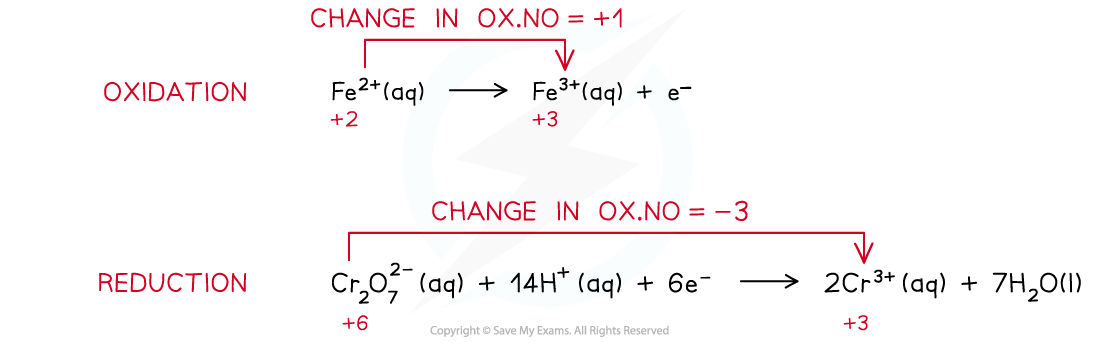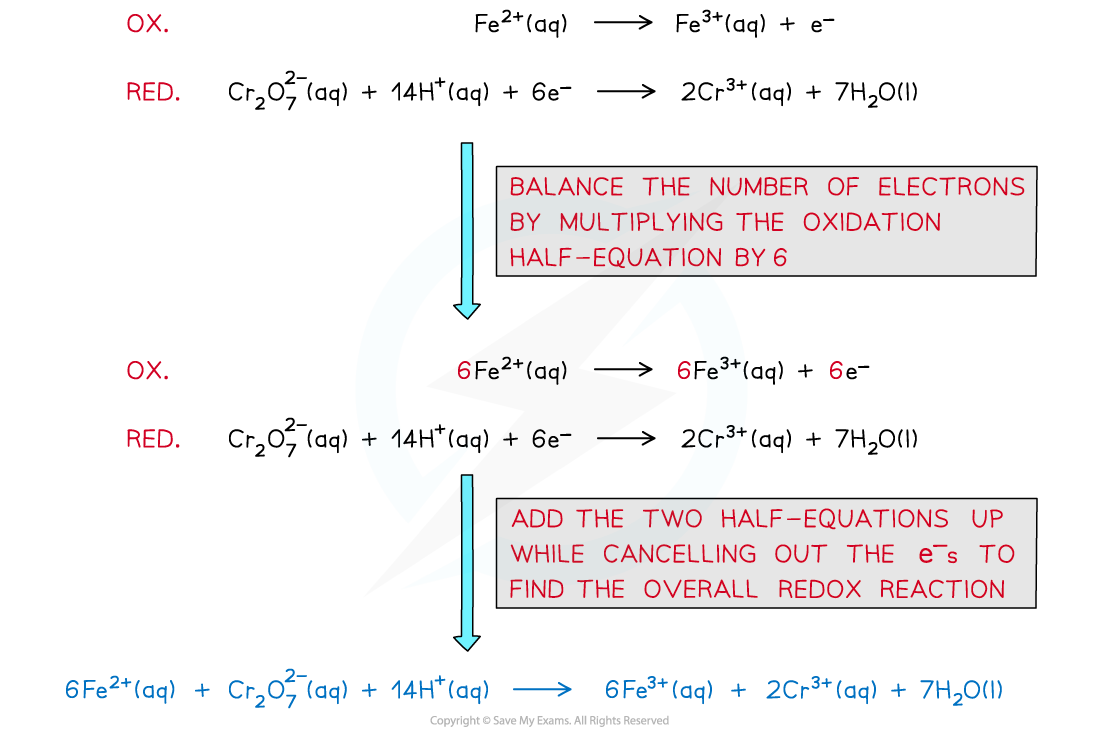# AQA A Level Chemistry复习笔记6.2.6 Redox Titrations

### Redox Titrations

• Redox titration involves an oxidising agent being titrated against a reducing agent
• Electrons are transferred from one species to another
• In acid-base titrations indicators are used to show the endpoint of a reaction; however redox titrations using transition metal ions naturally change colour when changing oxidation state, so indicators are not always necessary
• They are said to be 'self-indicating'
• Two common transition metal ion redox titrations are manganate(VII) and dichromate(VI) titrations

#### Manganate(VII) Titrations

• Potassium manganate(VII) is an oxidising agent and is a deep purple colour
• In acidic solutions it is reduced to the almost colourless manganese(II) ion (the ion is actually pink, but in low concentrations it is effectively colourless)
• The reduction equation for the manganate(VII) ion is

MnO4- (aq) + 8H+ (aq)  + 5e-   →   Mn2+ (aq)  + 4H2O (aq)

purple                                            colourless

• The potassium ion is a spectator ion so can be left out of the equations
• By convention the potassium manganate(VII) solution is placed in the burette, so that as it reacts with the reducing agent the solution becomes colourless
• At the endpoint the manganate(VII) ion becomes in excess so the first appearance of a permanent colour change marks the endpoint
• The colour seen is pink; if the colour is purple then you have overshot the endpoint and there is too much manganate(VII) in excess
• Analysis of iron in iron(II)sulfate tablets is typical manganate(VII) titration

#### Worked Example

A health supplement tablet contain iron(II)sulfate was analysed by titration. A tablet weighing 2.25 g was dissolved in dilute sulfuric acid and titrated against 0.100 mol dm-3 KMnO4 .The titration required 26.50 cm3 for complete reaction. Calculate the percentage by mass of iron in the table.

Step 1: Write the balanced equation for the reaction

oxidation: Fe2+ (aq)  →   Fe3+ (aq)  + e-

reduction: MnO4- (aq) + 8H+ (aq)  + 5e-   →   Mn2+ (aq)  + 4H2O (l)

overall: MnO4- (aq) + 8H+ (aq)  + 5Fe2+ (aq)   →   Mn2+ (aq)  + 4H2O (l) + 5Fe3+ (aq)

Step 2: Determine the amount of MnO4- used in the titration

moles of MnO4-  = 0.0265 dm3  x  0.100 mol dm-3 = 0.00265 mol

Step 3: Determine the amount of iron in the reaction

From the equation for the reaction we know the reacting ratio  MnO4- : Fe2+ = 1: 5

∴ moles of Fe2+ = 0.00265 mol MnO4- x 5 = 0.01325 mol

Step 4: Convert moles into mass of iron

Mass of iron = 0.01325 mol x 55.85 gmol-1 = 0.740 g

Step 5: find the percentage of iron in the tablet

∴ % Fe in the tablet = (0.740/ 2.25)  x 100 = 32.9%

#### Dichromate(VI) Titrations

• Potassium dichromate(VI), K2Cr2O7, is another oxidising agent used in redox titrations
• The oxidation state of the chromium changes from +6 to +3 in the reaction:

Cr2O7- (aq) + 14H+ (aq)  + 6e-   →   2Cr3+ (aq)  + 7H2O (l)

orange                                                  green

• From the half equation you can see that the reaction needs hydrogen ions so the solution must be acidified with excess dilute sulfuric acid
• The colour change is hard to see as the dichromate(VI) ion is pale orange and the chromium(III) ion is pale green
• To enhance the endpoint an indicator called diphenylaminesulfonate is used which turns from colourless to purple at the endpoint
• To obtain the balanced equation in the analysis of iron(II) first work out the redox changes in the half equations, then balance the number of electrons transferred:The steps in producing a balanced redox equation between iron(II) and dichromate(VI)

#### Exam Tip

Always show your working in redox titration problems as marks can be awarded for the steps even if the final answer is wrong.